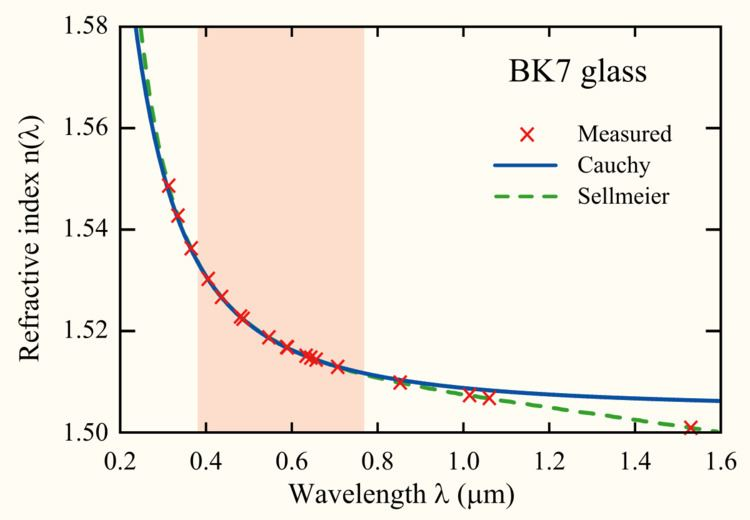# Cauchy's equation

Updated onCauchy's equation is an empirical relationship between the refractive index and wavelength of light for a particular transparent material. It is named for the mathematician Augustin-Louis Cauchy, who defined it in 1836.

## The equation

The most general form of Cauchy's equation is

n ( λ ) = B + C λ 2 + D λ 4 + ,

where n is the refractive index, λ is the wavelength, B, C, D, etc., are coefficients that can be determined for a material by fitting the equation to measured refractive indices at known wavelengths. The coefficients are usually quoted for λ as the vacuum wavelength in micrometres.

Usually, it is sufficient to use a two-term form of the equation:

n ( λ ) = B + C λ 2 ,

where the coefficients B and C are determined specifically for this form of the equation.

A table of coefficients for common optical materials is shown below:

The theory of light-matter interaction on which Cauchy based this equation was later found to be incorrect. In particular, the equation is only valid for regions of normal dispersion in the visible wavelength region. In the infrared, the equation becomes inaccurate, and it cannot represent regions of anomalous dispersion. Despite this, its mathematical simplicity makes it useful in some applications.

The Sellmeier equation is a later development of Cauchy's work that handles anomalously dispersive regions, and more accurately models a material's refractive index across the ultraviolet, visible, and infrared spectrum.

Topics